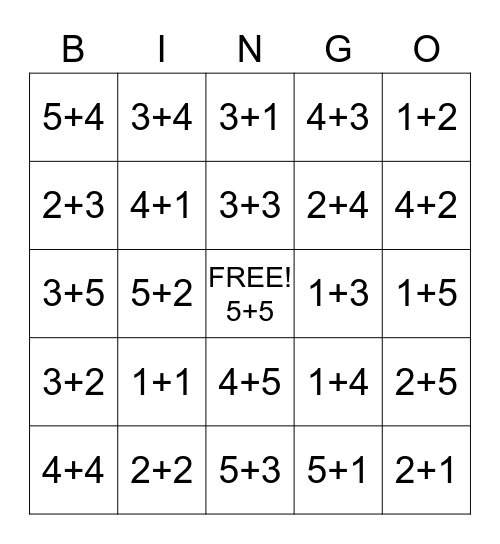This bingo card has a free space and 24 words: 1+1, 2+2, 3+3, 4+4, 1+2, 5+4, 2+3, 3+4, 1+3, 4+5, 2+4, 3+5, 1+4, 2+5, 4+1, 5+1, 1+5, 3+1, 4+2, 5+2, 2+1, 3+2, 4+3 and 5+3.

## Play Online